Download Presentation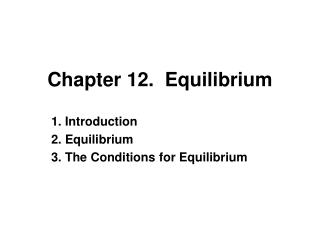Chapter 12. Equilibrium

# Chapter 12. Equilibrium - PowerPoint PPT Presentation

Chapter 12. Equilibrium. 1. Introduction 2. Equilibrium 3. The Conditions for Equilibrium. Introduction. Equilibrium. The linear momentum of its center of mass is constant. 2.   Its angular momentum about its center of mass, or about any other point, is also constant.I am the owner, or an agent authorized to act on behalf of the owner, of the copyrighted work described.
Download Presentation## Chapter 12. Equilibrium

An Image/Link below is provided (as is) to download presentation

Download Policy: Content on the Website is provided to you AS IS for your information and personal use and may not be sold / licensed / shared on other websites without getting consent from its author.While downloading, if for some reason you are not able to download a presentation, the publisher may have deleted the file from their server.

- - - - - - - - - - - - - - - - - - - - - - - - - - E N D - - - - - - - - - - - - - - - - - - - - - - - - - -
Presentation Transcript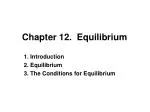### Chapter 12. Equilibrium

1. Introduction

2. Equilibrium

3. The Conditions for Equilibrium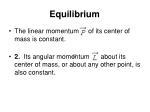Equilibrium
• The linear momentum of its center of mass is constant.
• 2.  Its angular momentum about its center of mass, or about any other point, is also constant.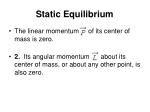Static Equilibrium
• The linear momentum of its center of mass is zero.
• 2.  Its angular momentum about its center of mass, or about any other point, is also zero.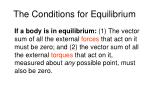The Conditions for Equilibrium

If a body is in equilibrium: (1) The vector sum of all the external forces that act on it must be zero; and (2) the vector sum of all the external torques that act on it, measured about any possible point, must also be zero.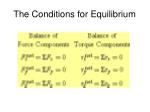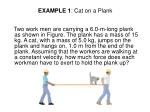EXAMPLE 1: Cat on a Plank

Two work men are carrying a 6.0-m-long plank as shown in Figure. The plank has a mass of 15 kg. A cat, with a mass of 5.0 kg, jumps on the plank and hangs on, 1.0 m from the end of the plank. Assuming that the workers are walking at a constant velocity, how much force does each workman have to exert to hold the plank up?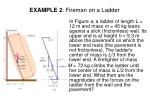EXAMPLE 2: Fireman on a Ladder

In Figure a, a ladder of length L = 12 m and mass m = 45 kg leans against a slick (frictionless) wall. Its upper end is at height h = 9.3 m above the pavement on which the lower end rests (the pavement is not frictionless). The ladder's center of mass is L/3 from the lower end. A firefighter of mass

M = 72 kg climbs the ladder until her center of mass is L/2 from the lower end. What then are the magnitudes of the forces on the ladder from the wall and the pavement?